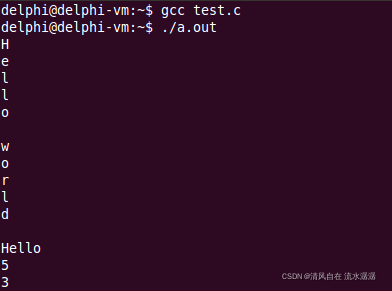﻿ C语言 详解字符串基础_C 语言_脚本之家
C 语言# C语言 详解字符串基础

## 一、字符串的概念

C 语言中没有字符串的概念

• C 语言中通过特殊的字符数组模拟字符串
• C 语言中的字符串是以 ‘\0’ 结尾的字符数组

## 二、字符数组与字符串

• 存储于程序的全局只读存诸区
• 本质为字符数组，编译器自动在结尾加上 ‘\0' 字符

```#include <stdio.h>

int main()
{
char ca[] = {'H', 'e', 'l', 'l', 'o'};
char sa[] = {'W', 'o', 'r', 'l', 'd', '\0'};
char ss[] = "Hello world!";
char* str = "Hello world!";

printf("%s\n", ca);
printf("%s\n", sa);
printf("%s\n", ss);
printf("%s\n", str);

return 0;
}```## 三、字符串字面量的秘密

• 字符串字面量的本质是一个数组
• 字符串字面量可以看作常量指针
• 字符串字面量中的字符不可改变
• 字符串字面量至少包含一个字符

"Hello World !”是一个无名的字符数组

```#include <stdio.h>

int main()
{
char b = "abc";
char c = *("123" + 1);
char t = *"";

printf("%c\n", b);
printf("%c\n", c);
printf("%d\n", t);

printf("%s\n", "Hello");
printf("%p\n", "World");

return 0;
}```## 四、字符串的长度

• 字符串的长度就是字符串所包含字符的个数
• 字符串长度指的是第一个 ‘\0’ 字符前出现的字符个数
• 通过 '\0' 结束符来确定字符串的长度
• 函数 strlen 用于返回字符串的长度

```#include <stdio.h>
#include <string.h>

int main()
{
char s[] = "Hello\0world";
int i = 0;

for(i=0; i<sizeof(s)/sizeof(char); i++)
{
printf("%c\n", s[i]);
}

printf("%s\n", s);

printf( "%d\n", strlen(s) );
printf( "%d\n", strlen("123") );

return 0;
}```s 代表字符串的话代表的就是 Hello，s 如果代表字符数组的话代表的就是 Hello\0world\0

## 五、小结

• C 语言中通过字符数组模拟字符串
• C 语言中的字符串使用 ‘\0’ 作为结束符
• 字符串字面量的本质为字符数组
• 字符串相关函数都依赖于结束符 ‘\0'